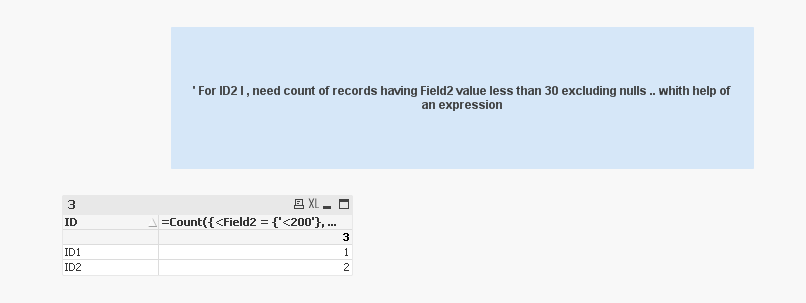# New to QlikView

Discussion board where members can get started with QlikView.

Valued Contributor

## Set expression Based on selection of multibox where a particular value should move to specific variable

Hi All ,

I have a scenario where in a Multibox I have four country , France , Germany , UK , US .Below is a set expression

Sum({<Value=varible1,Field1={xyz}>}Sale)+
Sum({<Value=varible2>},Field2={xyz}Sale)+
Sum({<Value=varible3>},Field3={xyz},Sale)+
Sum({<Value=varible4>},Field4={xyz},Sale)

My Requirement :

SCENARIO 1 : Single Select

if User selects France from Multibox then , France should go to variable 1 and return me value , but at same time set expression containing other variables should force return me 0

if user selects Germany then , Germany should go go to variable 2 and return me value , but at same time set expression containing other variables should force return me 0

SCENARIO 2 : Multiple Select

if User selects France and Germany from Multibox then France should go to variable 1 and Germany should go go to variable 2 and return me their sum , but at same time set expression containing other variables 3 and 4 should force return me 0

If user selects all four countries then variable  1 should take France , variable 2 should take Germany , variable 3 should take UK , 4 US

if user select France and UK then 1 should take France and variable 3 should take UK  while expression with variable 2 and 4 should return forced 0

Based of selection in multibox values should move to respective variable .

1 Solution

Accepted SolutionsMVP

## Re: Set expression Based on selection of multibox where a particular value should move to specific variable

Like this

=Count({<Field2 = {'<200'}, ID *= {'ID1'}>+<Field2 = {'<30'}, ID *= {'ID2'}>+<Field2 = {'<50'}, ID *= {'ID3'}>}ID)17 RepliesMVP

## Re: Set expression Based on selection of multibox where a particular value should move to specific variable

So is this question more relating to getting the right value into the variable? Would you be able to share a sample?

Valued Contributor

## Re: Set expression Based on selection of multibox where a particular value should move to specific variable

Yes ... Exactly .. Right value into variable ...  I am really sorry I cant share a sample , actually its based on live real data .MVP

## Re: Set expression Based on selection of multibox where a particular value should move to specific variable

May be like this for Variable1

SubField(Concat(DISTINCT Country, '|'), '|', 1)

and so on....

Valued Contributor

## Re: Set expression Based on selection of multibox where a particular value should move to specific variable

But will that force expression with other varible to yield 0 ? Actually that is also equally important and neededMVP

## Re: Set expression Based on selection of multibox where a particular value should move to specific variable

It might be much easier if you can share a sample to show how this can work....MVP

## Re: Set expression Based on selection of multibox where a particular value should move to specific variable

Do you really need to do this with variables. I may not understand your requirements exactly, but it looks like the native QV functionality should be able handle them.

Logic will get you from a to b. Imagination will take you everywhere. - A EinsteinMVP

## Re: Set expression Based on selection of multibox where a particular value should move to specific variable

I agreeMVP

## Re: Set expression Based on selection of multibox where a particular value should move to specific variable

I am not sure, what you are trying to achieve. As QlikView's default nature, if you select any country, qlikview will give you results for that country, also if you select multiple country, qlikview will give you result for two selected country. This is what we call it as AQL.

But to your requirement, you can do something like below

Variable1

=if(wildmatch(Concat(DISTINCT '|'&Country&'|',','),'*|France|*'),Only({<Country={'France'}>}Country),0)

Variable2

=if(wildmatch(Concat(DISTINCT '|'&Country&'|',','),'*|Germany|*'),Only({<Country={'Germany'}>}Country),0)

Variable3

=if(wildmatch(Concat(DISTINCT '|'&Country&'|',','),'*|UK|*'),Only({<Country={'UK'}>}Country),0)

Variable4

=if(wildmatch(Concat(DISTINCT '|'&Country&'|',','),'*|US|*'),Only({<Country={'US'}>}Country),0)

Sum({<Value={'\$(varible1)'},Field1={'xyz'}>}Sale)+

Sum({<Value={'\$(varible2)'},Field1={'xyz'}>}Sale)+

Sum({<Value={'\$(varible3)'},Field1={'xyz'}>}Sale)+

Sum({<Value={'\$(varible4)'},Field1={'xyz'}>}Sale)

Valued Contributor

Hi ,